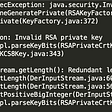# Solution in Python 3

`def kangaroo(x1, v1, x2, v2):    if x1 < x2 and v1 < v2:        return 'NO'    else:        if v1!=v2 and (x2-x1)%(v2-v1)==0:            return 'YES'         else:            return 'NO'`

# Explanation in Python 3

First, We check whether x1 is less than x2 and v1 is less than v2. If the condition is True then it is not possible for the two Kangaroos to be at the same location at the same time and hence we return ‘NO’.

This is because, when the first Kangaroo has a start at a preceding location as well as has a lower jump rate than the second Kangaroo then it is not possible for the first Kangaroo to catch up with the second Kangaroo.

In our program we represent it as:-

`if x1 < x2 and v1 < v2:        return 'NO'`

Else, If the speed of the Kangaroos are not equal to each other and the modulus of difference between the starting location of two Kangaroos and difference between the jump rate of two Kangaroos is zero then we return ‘YES’.

which is represented as:-

if v1!=v2 and (x2-x1)%(v2-v1)==0

To further explain the if statement, There is not a possibility of two Kangaroos to begin at the same location according to the problem thus at all times x1 is not equal to x2, thus if the jump rate of both Kangaroos are equal it is not possible for them to be at the same location at the same time. Therefore we check:-

v1!=v2

In the second part of our if statement, We check if the difference of distance i.e (x2-x1) and the difference of jump rate i.e (v2-v1) are multiples of each other. (If modulus of two numbers is zero then they are multiples of each other.) The condition in code is represented as:-

(x2-x1)%(v2-v1)==0

If both of the above conditions of if statements are True then it is possible for two Kangaroos to be at the same location at the same time and thus we return ‘YES’.

We represent above logic in our code in the following way:-

`else:     if v1!=v2 and (x2-x1)%(v2-v1)==0:         return 'YES'`

If the condition in the above if statement is False then it is not possible for the two Kangaroos to be at the same location at the same time, Hence we return ‘NO’

`else:      return 'NO'`

--

--

--

## More from Shounak Lohokare

pretending to teach is one of the best way to learn.

Love podcasts or audiobooks? Learn on the go with our new app.

## How to resolve RSA private key failed to load from pkcs12## Breaking the law of physics to become a developer## CNN anchor #ChrisCuomo was accused of sexual harassment Friday by a former female manager at ABC…## Run Azkaban Cluster on AWS## Git and GitHub (Part-2)## How to fix Python SSL CERTIFICATE_VERIFY_FAILED## Shounak Lohokare

pretending to teach is one of the best way to learn.

## Structured Query Language: an introduction## Python interview questions to know it must.## Constructor Type in Python